## General Question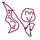# What is the value of .03 *.50?

Asked by milla101 (221) July 15th, 2009

What is the value of 0.03 *0.50 The answer is “0.015” Once again I’ve no idea how that comes about??? =S

Observing members: 0Composing members: 0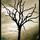If you multiply .03 and .5 you get .015 Come on these are easy!

BBSDTfamily (6834)“Great Answer” (0) Flag as…Easy on a calculator, sure. What’s the procedure?

milla101 (221)“Great Answer” (0) Flag as…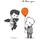0.5 = a half, so a half of 0.03 is 0.015. Say if you multiplied 0.03 by 1000, you’d have 30 and a half of 30 is 15 yeah? Divide 15 by a thousand and you get 0.015.

By the way, I’m happy to help and welcome to Fluther but it is not a website where people do your homework for you. Oh, I’m glad you’re asking for the procedure though :)

shrubbery (10321)“Great Answer” (3) Flag as…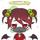0.03×0.5 can be changed to 0.03 / 2

ragingloli (50158)“Great Answer” (1) Flag as…Cheers for that, explained it perfectly.

milla101 (221)“Great Answer” (0) Flag as…By the way I didn’t mean to be rude or anything, I’m just warning you. You can certainly ask for help and a nudge but people here get testy if you ask them to do your homework for you. I can see you are not but are asking to be shown how to do it yourself so you can continue, so I apologise :)

shrubbery (10321)“Great Answer” (1) Flag as…No worries =)

milla101 (221)“Great Answer” (0) Flag as…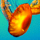The procedure is to start by multiplying as if the decimal point were not there. 0.03 * 0.50 => 3 * 50 = 150.

Then you add the number of decimal places in both terms and put that number of places in the result. In this case, they both have 2 decimal places, so you need 4 decimal places in the result. 150 => 0.0150.

Didn’t you take sixth grade math?

cwilbur (14219)“Great Answer” (2) Flag as…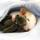It’s absolutely worthless.

ratboy (15167)“Great Answer” (0) Flag as…LoLz no actually I didn’t. =S

milla101 (221)“Great Answer” (0) Flag as…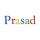0.03 * 0.50
=(3/100)*(5/10) ‘Get tens (or powers of tens) in denominators
=(3*5)/(100*10) ‘Separate numerator and denominator
=15/1000 ‘Proceed

prasad (3859)“Great Answer” (2) Flag as…There is a general method for determining where the decimal point goes. This problem can be used as an example.

..03 has the decimal point moved two places to the left from 3, which means that .03 = 3/(10*10). Similarly .50 has the decimal point moved two places to the left from 50 so .50 = 50/(10*10).

Then .03 * .50 = 3/(10*10) * 50/(10*10) = 3*50/(10*10*10*10) = 150/(10*10*10*10), which is 150 with the decimal point moved 4 times to the left, which is .0150, which is the same as .015.

In general, to multiply two numbers with decimal points, first multiply the numbers as if they did not have decimal points. Then add the number of times the decimal point had to be moved for each of the numbers and that tells how many places to move the decimal point in the answer.

LostInParadise (29758)“Great Answer” (0) Flag as…Yeah, as you said.
If you have difficulty for counting decimals, then first remove right-most zeros; e.g. 0.50 would then be 0.5.
Then, count the numbers to the right of decimal point. And add those many zeros in the tens in the denominator (0.5—>one count->10 in denominator, 0.03—>two count->100 in denominator, and so on).
Multiply all the terms in the numerator and denominator. Keep in mind that for multiplication of tens, just add number (count) of zeros and write 1 before (10*100—>one and two zeros, so total three zeros->1000).
Now, as you arrive with 15 divided by 1000, again count number of zeros in the denominator (three zeros here). The decimal point in the division would come those many digits left (start from right-most digit) from the number in the numerator. Add zero if the count is more than the number of digits in the numerator (as it is in this case, 15—>count is two and there are three zeros in denominator, start from right of 15, take first 5 (of 15), then 1(of 15), add one zero, and place the decimal point. So, the result is 0.015).
This technique works for as many numbers you want to multiply, two, three, or more.
Keep practising. All the best!

prasad (3859)“Great Answer” (0) Flag as…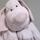I guess that since the equation states 0.03*0.50, the decimal accuracy is 2 digits.
Otherwise it could have stated 0.5 (neglecting accuracy) or 0.500 (more accuracy).

Accuracy of the result cannot exceed the accuracy of any of the factors so although the answers above are correct, it should actually be taken into account that there is a difference in multiplying by 0.50 versus dividing by two or multiplying by 0.5 .
Taking accuracy into account the answer to this calculation should be 0.02

whitenoise (14142)“Great Answer” (0) Flag as…@whitenoise: That’s true in science and engineering, but not in mathematics; and in science and engineering, significant digits count for more than decimal places.

cwilbur (14219)“Great Answer” (0) Flag as…@whitenoise “Accuracy of the result cannot exceed the accuracy of any of the factors…”
This may not always be correct, e.g. 0.3 * 0.4 = 0.12.
However, your answer is right with rounding it to two decimal places.

prasad (3859)“Great Answer” (0) Flag as…Well, in our math classes, we would loose points if we would not answer
0.30*0.4 = ? with: 0.30*0.4=0.12 -> 0.30*0.4=01.

We were instructed to keep accuracy in mind if we were confronted with formats with extra trailing zeros that indicate accuracy, or when the instruction explicitly asked for keeping accuracy in mind.

Maybe we had an overzealous teacher. It however stuck with me that 0.5, 0.50 and ½ are all of different accuracy.

whitenoise (14142)“Great Answer” (0) Flag as…@whitenoise: That’s an engineering approach to arithmetic, which isn’t wrong, but it’s not universal.

cwilbur (14219)“Great Answer” (0) Flag as…@cwilbur May also be that your math teachers teach math a little different from my European.

In our part of Europe, our engineers’ math is governed by the same rules of mathematics as that of the rest of us.

whitenoise (14142)“Great Answer” (0) Flag as…or